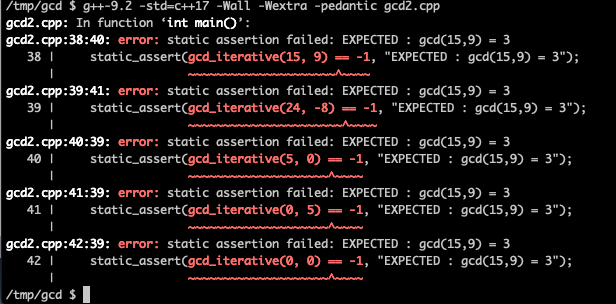# C++17 - find the greatest common divisor, gcd, of two or more integers

## Posted on October 25, 2019 by Paul

In this article, I will show you how to find the gcd - greatest common divisor of two or more integers with C++, by using two implementations of the classical Euclid algorithm. As a side note, the C++ standard library has a std::gcd function that can calculate the gcd of two numbers and I will also show you how to use the STL version. Implementing an algorithm from scratch is the best way to understand it, even though in practice one should use as much as possible the solution already provided by the language standard library.

There is also a video version of this tutorial:

By definition, the gcd of two or more integers, that are not all zero, is the largest positive integer that divides all these numbers. For example:

It is also useful to define:

Here are couple of properties of the gcd function:

The Euclid algorithm, or method, uses the fact that for two positive integers a and b:

Here is a worked example of using Euclid’s method to calculate gcd(15, 9):

finally, we can write:

Please note that, in the above calculations, we’ve used properties (1) and (4) of the gcd function.

At this point, we can write a C++ driver function that will ensure that we are doing the actual calculations only for positive integers:

Next, we’ll implement the iterative version of Euclid’s algorithm:

In the above function, we keep subtracting b from a and swapping a with b if a is less than b, until a becomes zero, at which point b contains the answer.

Line 4 in the above function calls a swap function. In principle we can use std::swap from the standard library, however I chose to implement my own version, since we are doing a from scratch version of gcd. Here is the implementation of my swap function:

Let’s test the above functions:

If you build the above program with a standard C++17 compiler you should get no error and all five tests should pass at compile time.

If you want to trigger error messages at compile time, you can modify the main function from the above code, e.g.:

This is what I see, if I build the above program with GCC 9.2 on a macOS machine:Next, let’s implement a recursive version of the Euclid algorithm. The driver function is basically the same, except that it will call euclid_gcd_recursive on line 13:

Next, we’ll implement the recursive version of Euclid’s algorithm:

Finally, let’s modify the main function by adding compile time tests for the recursive algorithm:

An optimization of the Euclid algorithm for calculating gcd is to replace the repetitive subtraction of b from a with the modulo operator. For example, this is the recursive version of the algorithm with the above optimization:

I’ve moved the optimized version of the above functions to file named gcd.h.

It is interesting to implement a gcd function that finds the greatest common divisor of a collection of integers, e.g.:

As promised, here is an example of using the standard library gcd implementation:

What about using std::gcd with a collection of integers? While you can use the above example with a loop over a vector, a simpler approach is to use std::reduce:

Please note that the above example will not compile with GCC 9! It works with VS 2019, Clang 9 and GCC 10.

You can find the complete source code on the GitHub repository for this article.